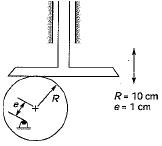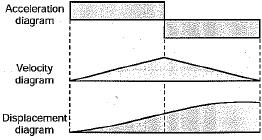Courses

# Test: Cams - 2

## 10 Questions MCQ Test Topicwise Question Bank for Mechanical Engineering | Test: Cams - 2

Description
This mock test of Test: Cams - 2 for Mechanical Engineering helps you for every Mechanical Engineering entrance exam. This contains 10 Multiple Choice Questions for Mechanical Engineering Test: Cams - 2 (mcq) to study with solutions a complete question bank. The solved questions answers in this Test: Cams - 2 quiz give you a good mix of easy questions and tough questions. Mechanical Engineering students definitely take this Test: Cams - 2 exercise for a better result in the exam. You can find other Test: Cams - 2 extra questions, long questions & short questions for Mechanical Engineering on EduRev as well by searching above.
QUESTION: 1

### The angle between the line of stroke (line of motion of the follower) and the normal to the pitch curve at any point, is referred to as

Solution:

The pressure angle, representing the steepness of the cam profile, is the angle between the normal to the pitch Curve at a point and the direction of the follower motion. If varies in magnitude at all instants of the follower motion. A high value of the maximum pressure angle is not desired as it might jam the follower in the bearings.

QUESTION: 2

### The pressure angle of a cam depends upon 1. lift and type of the follower motion 2. angle of ascent 3. offset between center line Which of the statements made above are correct?

Solution:

The pressure angle of a cam depends upon
- angle of ascent
- angle of dwell
- angle of descent
- angle of action
- offset between center line

QUESTION: 3

### Why is an offset provided in a radial cam - translating follower mechanism?

Solution:
QUESTION: 4

Identify the nature of motion in a cam - roller followers system that leads to lowest jerk

Solution:
QUESTION: 5

During the dwell period of cam, the follower

Solution:
QUESTION: 6

All of the followings are correct, except

Solution:

In a cam follower system, pitch joint is the point on the pitch curve at which the pressure angle is maximum.

QUESTION: 7

Pitch point on a cam is

Solution:
QUESTION: 8

Which of the following motion of cam roller - follower leads to the lowest jerk?

Solution:
QUESTION: 9

An eccentric circular cam of radius 10 cm as shown below rotates at a constant angular speed of 2 rad/s, The eccentricity is 1 cm. The maximum translational speed of the flat face follower isSolution:

Maximum speed = eωsinθ
= 1 x 2 x 1 = 2 cm/sec

QUESTION: 10

In a plate cam mechanism with reciprocating roller follower, the follower has a constant acceleration in the case of

Solution:

For uniform acceleration and retardation the velocity of the follower must change at a constant rate and hence the velocity diagram of the follower consists of sloping straight lines. The velocity diagram represents everywhere the slope of the displacement diagram, the latter must be a curve whose slope changes at a constant rate. Hence the displacement diagram consists of double parabola.